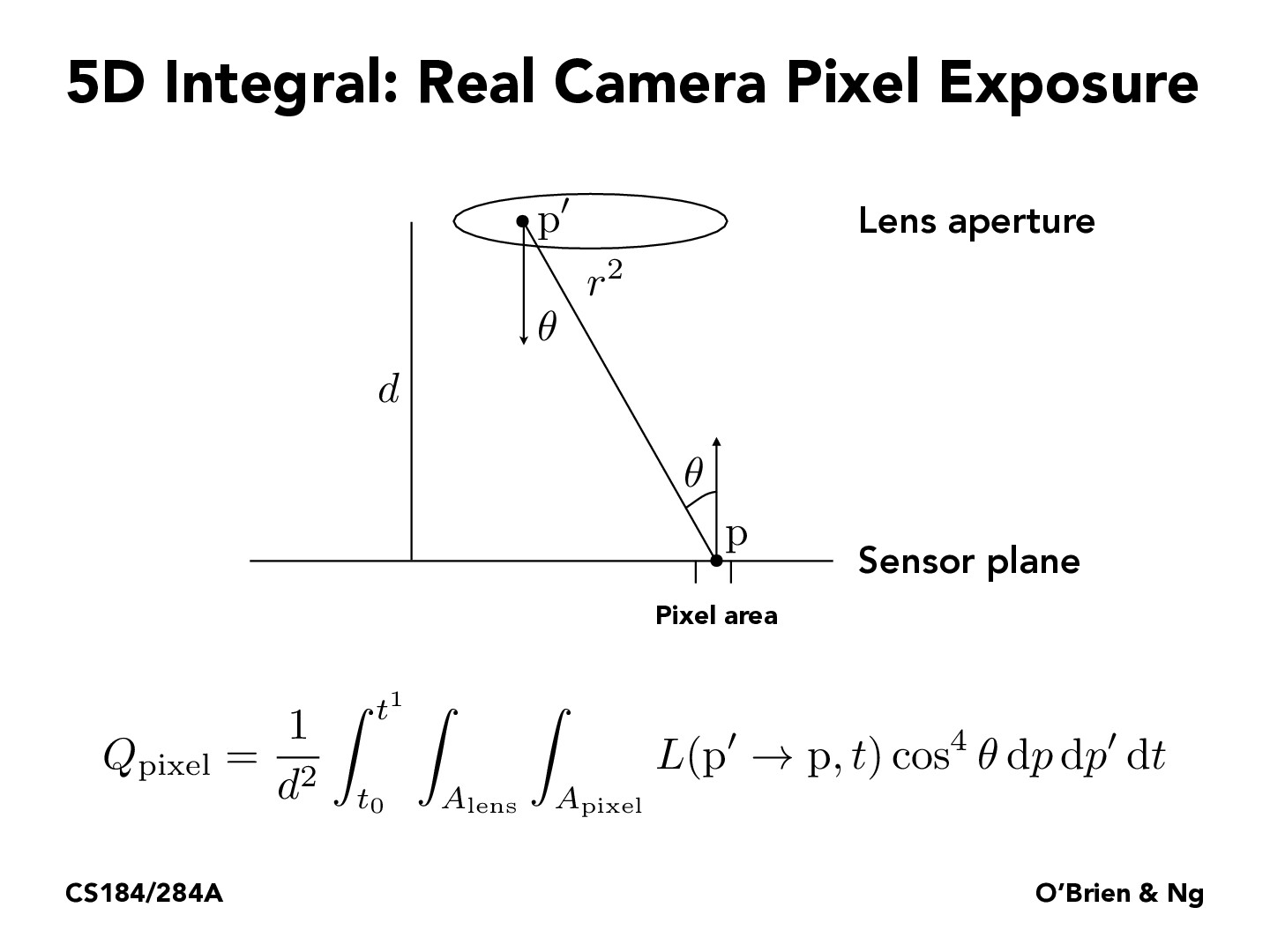Lecture 12: Monte Carlo Integration (7)rsha256

Does $p'\to p$ means the vector formed from point $p'$ to point $p$?

And that $L$ takes in a vector as its first parameter and a scalar $t$ as its second parameter, and returns a scalar?starptr

I believe $t$ is a vector, since $L$ refers to surface radiance, and $p^\prime \rightarrow p$ refers to the vector $p - p^\prime$

You must be enrolled in the course to comment